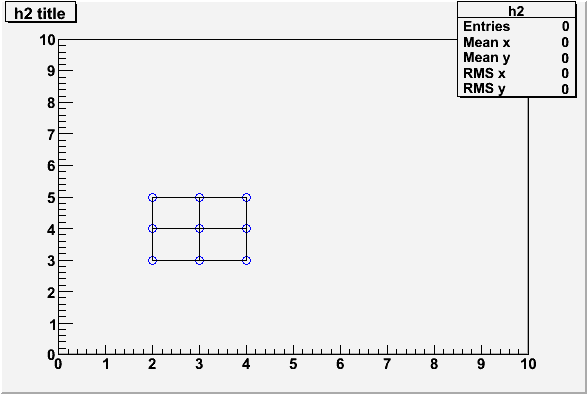# How to draw TLine use loop?

[code]{
TCanvas *c1 = new TCanvas(“c1”, “c1”,1);
TH2F *h2 = new TH2F(“h2”,“h2 title”,10,0,10,10,0,10);
h2->Draw();

TFile *f=new TFile(“data.root”,“RECREATE”);
TTree *t=new TTree(“T”,“data from ascii file”);
Int_t x,y;
t->Branch(“x”,&x,“x/I”);
t->Branch(“y”,&y,“y/I”);

UShort_t r,c;
for(r=2;r<=4;r++)
{
x=r;
for(c=3;c<=5;c++)
y=c;
printf("%d %d\n",x,y);
t->Fill();
}

for(x=2;x<=4;x++)
{
t->SetMarkerColor(4);
t->SetMarkerStyle(4);
for(y=3;y<=5;y++)
{
t->Draw(Form("%d:%d",y,x),"",“same”);
[color=blue] TLine a(x,3,x,y);
a.Draw(“same”);[/color]

``````       }
}
``````

c1->Modified();
}[/code]
In fact ,i want to draw c1.gif, but the programm can’t draw it

and i think use TLine is difficult when draw too square

please tell me how to draw it using other way?
i read the users-guide , but i find use TBox is also difficult,
thanks!``````{
TCanvas *c1 = new TCanvas("c1", "c1",1);
TH2F *h2 = new TH2F("h2","h2 title",10,0,10,10,0,10);
h2->Draw();

TFile *f=new TFile("data.root","RECREATE");
TTree *t=new TTree("T","data from ascii file");
Int_t x,y;
t->Branch("x",&x,"x/I");
t->Branch("y",&y,"y/I");

UShort_t r,c;
for (r=2;r<=4;r++) {
x=r;
for(c=3;c<=5;c++)
y=c;
printf("%d %d\n",x,y);
t->Fill();
}

TLine *a = new TLine();
for (x=2;x<=4;x++) {
t->SetMarkerColor(4);
t->SetMarkerStyle(4);
for (y=3;y<=5;y++) {
t->Draw(Form("%d:%d",y,x),"","same");
a->DrawLine(x,3,x,y);
}
}
}``````